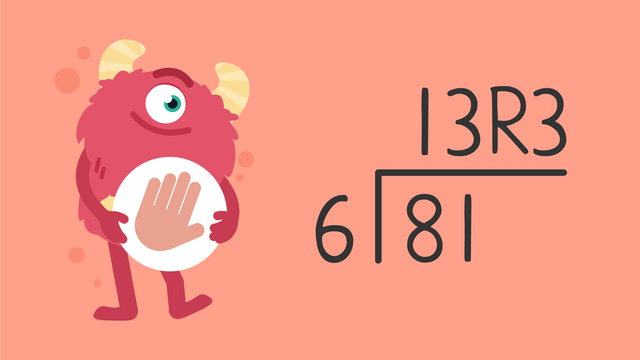# Long Division with Remainders—Let's Practice!Rating

Ø 5.0 / 1 ratings
The authorsTeam Digital
Long Division with Remainders—Let's Practice!
CCSS.MATH.CONTENT.4.NBT.B.6

## Basics on the topicLong Division with Remainders—Let's Practice!

Today we are practicing long division with remainders with Razzi! This video contains examples to help you further practice and grow confident in this topic.

### TranscriptLong Division with Remainders—Let's Practice!

Razzi says get these items ready (...) Because today we're going to practice... Long Division with Remainders. It's time to begin! Solve four hundred eight divided by five. Pause the video to work on the problem (...) and press play when you are ready to see the solution! First, five does not go into four so we look at forty,(...) five goes into forty eight times,(...) and forty minus forty is zero. Next, bring down the eight,(...) five goes into eight one time,(...) and eight minus five is three. Five goes into four hundred eight... eighty-one times, with three remaining. Did you also get eighty-one remainder three? Let's try one more! Solve six thousand seven hundred thirty-three divided by seven. Pause the video to work on the problem (...) and press play when you are ready to see the solution! First, seven does not go into six,(...) so we look at sixty-seven,(...) seven goes into sixty-seven nine times,(...) and sixty-seven minus sixty-three is four. Next, bring down the three,(...) seven goes into forty-three six times,(...) and forty-three minus forty-two is one. Next, bring down the last three,(...) seven goes into thirteen one time,(...) and thirteen minus seven is six. Seven goes into six thousand seven hundred thirty-three... nine hundred sixty-one times with six remaining. Did you also get nine hundred sixty-one remainder six? Razzi had so much fun practicing with you today! See you next time!

## Long Division with Remainders—Let's Practice! exercise

Would you like to apply the knowledge you’ve learned? You can review and practice it with the tasks for the video Long Division with Remainders—Let's Practice!.
• ### Solve 164 ÷ 3 with Razzi.

Hints

Follow the steps of long division until you have no more digits to bring down and are left with a remainder.

The remainder is what is left over and goes next to the quotient.

Start by dividing the first digit by the divisor, which is 3. However, since 3 cannot go into 1, we should look at the next two digits, which are 16. How many times does 3 go into 16?

Solution

164 ÷ 3 = 54 R2

• ### What is the remainder?

Hints

The remainder is what is left after going through all the steps of long division.

41÷5 =8R1 because 8 times 5 is 40 with 1 left over.

So, 1 is the remainder.

Solution

The solution for the problem is above. Here is one example of how you can solve these problems.

• 41÷5 = 8 R?
First, how many times does 5 go into 41? 8 times without going over so 8 times 5 equals 40. Then do 40 minus 41, what is left? 1! 1 is the remainder.

• ### Solve 5,783 $\div$ 8.

Hints

Can the divisor go into the first digit of the dividend? Can 8 go into 5?

Here are steps to solving a long division problem. You'll repeat until you have found a remainder.

Solution

The quotient and remainder of 5,783 $\div$ 8 = 722 R7.

• 8 goes into 57 7 times (to make 56)
• 8 goes into 18 2 times (to make 16)
• 8 goes into 23 2 times (to make 16)
• 7 remains.

• ### Solve the division problem.

Hints

Start by dividing the first digit by the divisor, which is 6. However, since 6 cannot go into 2, look at the first two digits, which makes 28. How many times does 6 go into 28?

Follow the steps of long division:

• Divide
• Multiply
• Subtract
• Bring down
• Repeat

Solution

28,371 ÷ 6 = 4,728 R3

• ### Solve the division problem.

Hints

If the divisor (5) cannot go into the first digit 3, look how many times 5 can go into 32.

Work through the steps of long division until there are no other numbers to bring down. The left over number will your remainder.

Solution

The quotient and remainder of 327 ÷ 5 = 65 R2.

• ### Which equation has the largest remainder?

Hints

Find the remainders of each division problem and sort in order from largest to smallest based on the remainders.

Here are the steps for long division.

Solution

Here is correct order based on the remaining eggs. Above is an example of how you can solve.

• 381÷ 12 = 31 R9
• 90 ÷12 = 7 R6
• 159÷12 = 13 R3
• 278÷12 = 23 R2